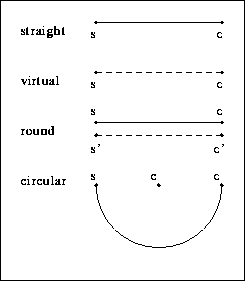Previous: Geometric Model Up: 3D-Modeling for the Analysis of Range Next: Surfaces

# Edges

At the moment four types of edges are used. They are very common and there exist methods to detect them in range data.Figure 6: Four types of edges

1. Straight Edges are easy to detect and easy to describe. They are the basic constituents of polyhedrical models. A Straight Edge is defined by start and end vertex. Derived parameters are length and orientation.

2. Virtual Edges were introduced because of syntactical reasons. They can be used to define edges which are too complex to define. So the meaning is:
• There is an edge but I do not know how to describe it.
• If you find something in the image, do not use it.
The second use for them is to add internal contours like drill-holes. Drill-holes are modeled because they are very characteristic and thus useful for the object recognition.

3. Round Edges are straight surface boundaries plus information about the neighborhood. They are a way to describe bends or flexures. Such structures are common in industrial environments (Figure 7).Figure 7: Example for the edge type Round Edge. Typical industrial objects and a corresponding model.

Round Edges are links between two surface patches, where we are not able to describe the interspace. But if we do no want to lose the information of those two surfaces being neighbors, we have to define an edge that consists of three parts:

1. the boundary of the surface,
2. the boundary of the neighbor surface

There are only straight Round Edges, so the linked plane surfaces normally have a line of intersection.

4. Circular Edges are needed to describe cylindrical surfaces or drill-holes in surfaces.

As the used tools are able to compute center, radius and orientation of circles it is useful not to approximate circles by polygons. Circular Edges are represented by start and end vertex plus center. Circle parameters like radius and orientation are derived from this data.

An edge has two independent characteristics:

1. geometric structure: straight, circular, spline, ...
2. visibility: not visible, visible, detectable.

edgegeometryvisibility
Straight Edgestraightvisible or detectable
Virtual Edgestraightnot visible
Round Edgestraightdetectable
Circular Edgecircularvisible or detectable
Table 1: Geometry and visibility of the four types of edges

As some combinations between geometric structure and visibility make no sense, it is not useful to model all of them. In case of the straight edges the meaning and also the structure of an edge is different for each type of visibility (table 1). Not visible circular edges make no difference to the straight Virtual Edge, because the geometry of invisible edges does not matter.

Round edges with circular geometry may be a useful addition, but as there was no example object with this characteristic, this edge type is not defined yet. It is very easy to add this edge type to the model, if necessary. So this is an example for the advantage of the modular modeling concept.

A special problem for the object recognition is the visibility of surface boundaries. The transition from a surface patch to the rounded intersection may be very smooth, see Figure 7. A similar edge is E4-E5 in Figure 8 where a cylinder patch turns into a plain patch. Although those edges are not visible in grey images, they are detectable in range data as they mark the border between surfaces of different characteristics. A problem is that they may be very inaccurate. Their position may depend on the point of view.Previous: Geometric Model Up: 3D-Modeling for the Analysis of Range Next: Surfaces

Last updated 1997/07/11, © Jochen Duckeck